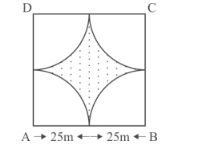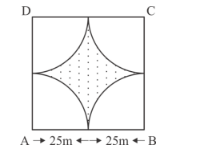# Four cows are tethered at four corners of a square plot of side 50 m,Question:

Four cows are tethered at four corners of a square plot of side 50 m, so that they just cannot reach one another. What area will be left ungrazed? (Fig. 15.66)Solution:

It is given that four cows are tethered at four corner of square ABCD. We have to find the area of plot that will left ungrazed.Let the side of square is a.

a = 25 + 25 m = 50 m

Area of square $=a^{2}$

$=50 \times 50$

$=2500 m^{2}$

Area of quadrant inside square $=\frac{1}{4} \pi r^{2}$

$=\frac{1}{4} \times \frac{22}{7} \times 25 \times 25$

$=491.07 \mathrm{~m}^{2}$

Area of shaded region $=$ Area of square $-4 \times$ Area of quadrant

$=2500-4 \times 491.07$

$=2500-1964.28$

$=535.71 \mathrm{~m}^{2}$